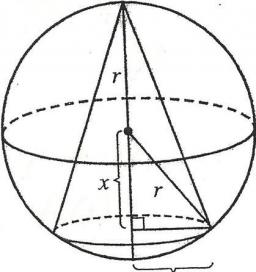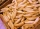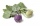# Sphere and cone

Within the sphere of radius G = 33 cm inscribe the cone with the largest volume. What is that volume, and what are the dimensions of the cone?

Correct result:

r =  31.11 cm
h =  44 cm
V =  44602.24 cm3

#### Solution:

$G = 33 \ cm \ \\ V = \dfrac13 \pi r^2 h \ \\ h = G + x = 33 +x \ \\ G^2 = x^2+r^2 \ \\ r^2 = G^2 - x^2 \ \\ V = \dfrac13 \pi (G^2 - x^2)(G+x) \ \\ V = \dfrac13 \pi (G^3 + G^2x - Gx^2 - x^3) \ \\ \ \\ V' = \dfrac13 \pi (G^2 - 2Gx - 3x^2) \ \\ V'=0 \ \\ G^2 - 2Gx - 3x^2=0 \ \\ -3x^2 -66x +1089 =0 \ \\ 3x^2 +66x -1089 =0 \ \\ \ \\ a=3; b=66; c=-1089 \ \\ D = b^2 - 4ac = 66^2 - 4\cdot 3 \cdot (-1089) = 17424 \ \\ D>0 \ \\ \ \\ x_{1,2} = \dfrac{ -b \pm \sqrt{ D } }{ 2a } = \dfrac{ -66 \pm \sqrt{ 17424 } }{ 6 } \ \\ x_{1,2} = \dfrac{ -66 \pm 132 }{ 6 } \ \\ x_{1,2} = -11 \pm 22 \ \\ x_{1} = 11 \ \\ x_{2} = -33 \ \\ \ \\ \text{ Factored form of the equation: } \ \\ 3 (x -11) (x +33) = 0 \ \\ \ \\ \ \\ h = G + x_1 = 33 + 11 = 44 \ cm \ \\ r = \sqrt{ G^2 - x_1^2 } = 31.11 \ \text{cm} \ \\$
$V = \dfrac13 \pi r^2 h = 44602.24 \ \text{cm}^3$

Our quadratic equation calculator calculates it.We would be pleased if you find an error in the word problem, spelling mistakes, or inaccuracies and send it to us. Thank you!

Showing 1 comment:Dr Math
that's very mind blowingTips to related online calculators
Looking for help with calculating roots of a quadratic equation?
Pythagorean theorem is the base for the right triangle calculator.

#### You need to know the following knowledge to solve this word math problem:

We encourage you to watch this tutorial video on this math problem:

## Next similar math problems:

• TV competitionIn the competition, 10 contestants answer five questions, one question per round. Anyone who answers correctly will receive as many points as the number of competitors answered incorrectly in that round. One of the contestants after the contest said: We g
• Secret treasureScouts have a tent in the shape of a regular quadrilateral pyramid with a side of the base 4 m and a height of 3 m. Determine the radius r (and height h) of the container so that they can hide the largest possible treasure.
• Paper boxHard rectangular paper has dimensions of 60 cm and 28 cm. The corners are cut off equal squares and the residue was bent to form an open box. How long must be side of the squares to be the largest volume of the box?
• Derivative problemThe sum of two numbers is 12. Find these numbers if: a) The sum of their third powers is minimal. b) The product of one with the cube of the other is maximal. c) Both are positive and the product of one with the other power of the other is maximal.
• Curve and lineThe equation of a curve C is y=2x² -8x+9 and the equation of a line L is x+ y=3 (1) Find the x co-ordinates of the points of intersection of L and C. (2) Show that one of these points is also the stationary point of C?
• PagansJano and Michael ate pagans. Jano ate three more than Michael. The product of their counts (numbers) is 180. How many pagans did each of them eat?
• StaircaseOn a staircase 3.6 meters high, the number of steps would increase by 3 if the height of one step decreased by 4 cm. How high are the stairs?
• The woodenThe wooden block measures 12 cm, 24 cm, and 30 cm. Peter wants to cut it into several identical cubes. At least how many cubes can he get?
• KohlrabiesThe price of one kohlrabi increased by € 0.40. The number of kohlrabies that a customer can buy for € 4 has thus decreased by 5. Find out the new price of one kohlrabi in euros.
• Area and perimeter of rectangleThe content area of the rectangle is 3000 cm2, one dimension is 10 cm larger than the other. Determine the perimeter of the rectangle.
• Isosceles triangleIn an isosceles triangle ABC with base AB; A [3,4]; B [1,6] and the vertex C lies on the line 5x - 6y - 16 = 0. Calculate the coordinates of vertex C.
• Dimensions of the trapezoidOne of the bases of the trapezoid is one-fifth larger than its height, the second base is 1 cm larger than its height. Find the dimensions of the trapezoid if its area is 115 cm2
• Integer sidesA right triangle with an integer length of two sides has one leg √11 long. How much is its longest side?
• The cylinderThe cylinder has a surface area of 300 square meters, while the height of the cylinder is 12 m. Calculate the volume of this cylinder.
• Lookout towerHow high is the lookout tower? If each step was 3 cm lower, there would be 60 more of them on the lookout tower. If it was 3 cm higher again, it would be 40 less than it is now.
• SummandsWe want to split the number 110 into three summands so that the first and the second summand are in the ratio 4: 5, and the third with the first are in ratio 7: 3. Calculate the smallest of the summands.
• Circle and squareAn ABCD square with a side length of 100 mm is given. Calculate the radius of the circle that passes through the vertices B, C and the center of the side AD.